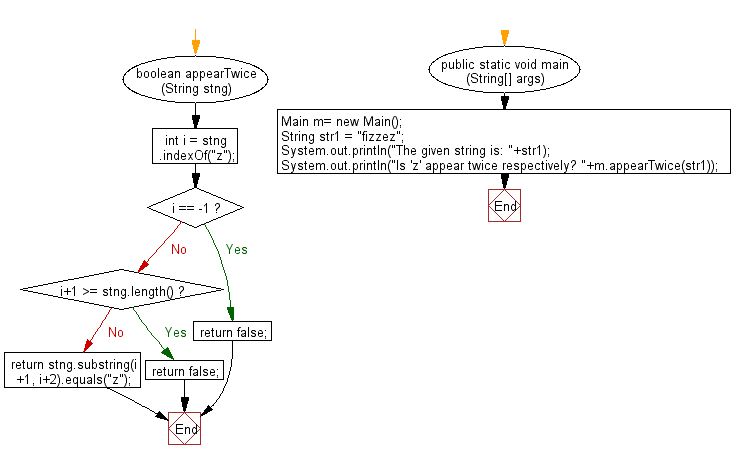﻿ Java exercises: Check whether the first instance of a given character is immediately followed by the same character in a given string - w3resource# Java String Exercises: Check whether the first instance of a given character is immediately followed by the same character in a given string

## Java String: Exercise-98 with Solution

Write a Java program to check whether the first instance of a given character is immediately followed by the same character in a given string.

Sample Solution:

Java Code:

``````import java.util.*;
public class Main
{
boolean appearTwice(String stng)
{
int i = stng.indexOf("z");
if (i == -1) return false;
if (i+1 >= stng.length()) return false;
return stng.substring(i+1, i+2).equals("z");
}

public static void main (String[] args)
{
Main m= new Main();
String str1 =  "fizzez";
System.out.println("The given string is: "+str1);
System.out.println("Is 'z' appear twice respectively? "+m.appearTwice(str1));
}
}
``````

Sample Output:

```The given string is: fizzez
Is 'z' appear twice respectively? true
```

Pictorial Presentation:Flowchart:Java Code Editor:

Improve this sample solution and post your code through Disqus

What is the difficulty level of this exercise?

﻿

## Java: Tips of the Day

Count the number of occurrences of a char in a String:

```int count = StringUtils.countMatches("a.b.c.d", ".");
```

Ref: https://bit.ly/2OTy6sG